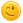# You will be given 3 integers as input. The inputs may or may not be different from each other.

You will be given 3 integers as input. The inputs may or may not be
different from each other.

You have to output 1 if all three inputs
are different from each other,
and 0 if any input is repeated more
than once.

Input
-----
Three integers on three lines.

Output
------
1 if the three inputs are different from each other
, 0 if some input is repeated more than once

+1 vote
answered Aug 5, 2019 by (1,600 points)

#include <stdio.h>
void main()
{
int a=0, b=0, c=0;
printf("%c",(a==b)?'0':(c==a)?'0':(c==b)?'0':'1',scanf("%d%d%d",&a,&b,&c));
}

Try this one.have used the ternary operator for deciding within the printf function combined with scanf to get the numbers.

answered Aug 22, 2019 by Rohit
#include <stdio.h>
int main() {
int a,b,c,x,y,z,w;
printf("enter three integers:");
scanf("%d%d%d",&a,&b,&c);
x=(a==b);
y=(b==c);
z=(a==c);
w=!(x+y+z);
printf("the value of w is=%d",w);
return 0;
}
answered Aug 22, 2019 by Suhas Gowda K R
//java program

public class Main

{

public static void main(String[] args) {

int x=Integer.parseInt(args);

int y=Integer.parseInt(args);

int z=Integer.parseInt(args);

if(x!=y && y!=z && z!=x)

return 1;

else if(x==y || y==z || z==x)

return 0;

}

}
answered Sep 2, 2019 by (550 points)
#include<stdio.h>

#include<conio.h>

void main()

{

int a,b,c;

scanf("%d%d%d",&a,&b,&c);

if((a!=b) && (b!=c) && (a!=c))

printf("1");

else

printf("0");

getch();

}
answered Sep 3, 2019 by (140 points)
#include <stdio.h>

int main()
{
int x,y,z;
printf("Enter values:");
scanf("%d\n%d\n%d",&x,&y,&z);
if(x==y||y==z||x==z)
printf("0");
else
printf("1");

return 0;
}
answered Sep 4, 2019 by Utkarsh Anand

# PYTHON 3 CODE#
import sys
numbers= list(map(int, input().split()))
for i in numbers:
if numbers.count(i)>1:
print("0")
sys.exit(0)
print("1")

commented Sep 7, 2019 by (110 points)
using conditional statements
include <stdio.h>

int main()
{
int a,b,c;
printf("enter three numbers :");
scanf("%d%d%d",&a,&b,&c);
if(a==b&&a==c)
{
printf("0");
}
else if(b==a&&b==c)
{
printf("0");
}
else if(c==a&&c==b)
{
printf("0");
}
else
{
printf("1");
}
getch();
answered Sep 6, 2019 by anonymous
import java.util.*;

public class Main

{

public static void main(String[] args) {

Scanner sc=new Scanner(System.in);

int a=sc.nextInt();

int b=sc.nextInt();

int c=sc.nextInt();

if(a!=b && b!=c && c!=a)

System.out.println("1 different");

if(a==b || b==c || c==a)

System.out.println("0 repeated");

}

}
answered Sep 9, 2019 by anonymous
#include<bits/stdc++.h>

using namespace std;

int main()

{
long long a,b,c;  // we input three number

cin >> a >> b >> c;

if (a!=b && a!=c && b!=c)

{
cout<<1;

}

else cout<<"0";